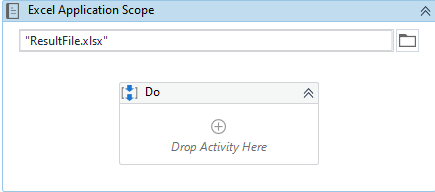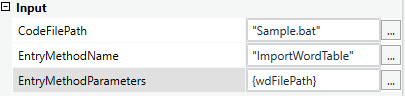# Import Datatable From Word Document To Excel File

UiPath provides us the flexibility to export the data from the word document to excel file, here one more exciting way to achieve the result.

1. You can create a .bat file using the below code
``````Sub ImportWordTable(wdFileName)

Dim wdDoc As Object
Dim tableNo As Integer 'table number in Word
Dim iRow As Long 'row index in Excel
Dim iCol As Integer 'column index in Excel
Dim resultRow As Long 'from which row result should be written
Dim tableStart As Integer
Dim tableTot As Integer

On Error Resume Next

ActiveSheet.Range("A1:AZ").ClearContents

Set wdDoc = wdApp.Documents.Open(Filename:=wdFileName, AddToRecentFiles:=False, Visible:=False)
Set wdDoc = GetObject(wdFileName)
With wdDoc
tableNo = wdDoc.tables.Count
tableTot = wdDoc.tables.Count

resultRow = 1'from where the result should be written in the excel

For tableStart = 1 To tableTot
With .tables(tableStart)
'copy cell contents from Word table cells to Excel cells
For iRow = 1 To .Rows.Count
For iCol = 1 To .Columns.Count
Cells(resultRow, iCol) = WorksheetFunction.Clean(.cell(iRow, iCol).Range.Text)
Next iCol
resultRow = resultRow + 1
Next iRow
End With
resultRow = resultRow + 1
Next tableStart
End With

End Sub
``````
1. Use Excel Application Scope activity to import the data from Word Document to the Excel and in the Do section use either Invoke VBA activity to run that bat file.2. Path the file Path into the CodeFilePath, method name means the sub function name of the above code in EntryMethodName and the parameters in the EntryMethodParameters which is optional if you create the parameterized code than only not to pass the parameter else you can skip it.This way the whole datatable from the Word Document would be imported to the excel.

4 Likes